# Problem 52834. Easy Sequences 32: Almost Pythagorean Triples

An Almost Pythagorean Triple (abbreviated as "APT'), is a set of 3 integers in which square of the largest element, which we will call as its 'hypotenuse', is 1 less than the sum of square of the smaller elements (shorter sides). This means that if c is the hypotenuse and a and b are the shorter sides,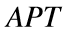, satisfies the following equation: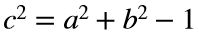where: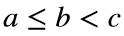The smallestis the triple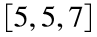, with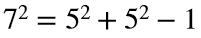and perimeter (the sum of the 3 elements) of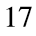. Some researchers consider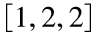as the smallest, but here, we will only look at's where the hypotenuse is "strictly" greater than the other shorter sides. Other examples of's are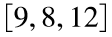, and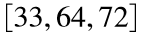.
Unfortunately, unlike Pythagorean Triples, a 'closed formula' for generating all possible's, has not yet been discovered, at the time of this writing. For this exercise, we will be dealing with's with a known ratio between the hypotenuse and the shortest side: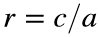.
Given the value of r, find the perimeter of thewith the r-th smallest perimeter. For example for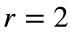, that is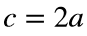, the smallest perimeter is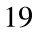for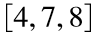, while the second (r-th) smallest perimeter is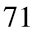, for thewith dimensions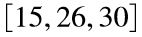. For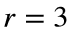, the third smallest perimeter is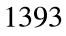for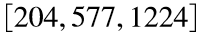.
The output can be very large, so please present only the last 12 digits if the number of digits of the perimeter exceeds 12.

### Solution Stats

40.0% Correct | 60.0% Incorrect
Last Solution submitted on Oct 07, 2022

### Community Treasure Hunt

Find the treasures in MATLAB Central and discover how the community can help you!

Start Hunting!